Definitions of Square Dance Calls and Concepts
Exchange The Box [C3A]Index -->  Plus  |  A1  |  A2  |  C1  |  C2  |  C3A  |  C3B  |  C4  |  NOL  |
Definitions (Text Only) -->  Plus  |  A1  |  A2  |  C1  |  C2  |  C3A  |  C3B  |  C4  |  NOL  |
 Find call:

 \$B8@8l(B2 x 4 \$B\$+\$i!%(B

Split Circulate \$B\$r9g7W(B 4 \$B2s9T\$\$\$^\$9\$,(B, \$BCf\$r8~\$\$\$?(B Center \$B\$H\$J\$C\$?\$H\$-\$O\$N(B box \$B\$X(B cross over \$B\$7\$^\$9(B)\$B!%(BExchange The Box \$B\$O(B 4 \$B\$D\$N%Q!<%H\$KJ,\$1\$i\$l\$^\$9!%(B

\$BB>\$N(B box \$B\$X(B cross \$B\$9\$k\$H\$-\$NDL\$jJ}\$G\$9\$,(B, \$B\$^\$@<+J,\$N%*%j%8%J%k\$N(B box \$B\$K\$\$\$k?M\$N30B&\$rDL\$j\$^\$9!%Cf\$r8~\$\$\$?(B Centers \$B\$,(B Exchange \$B\$N%j!<%@!<\$H\$J\$j(B, \$BIaDL\$N>l9g(B box \$B\$NB>\$N?M\$O\$=\$N?M\$K\$D\$\$\$F9T\$/\$3\$H\$,\$G\$-\$^\$9!%(B

\$BJ?9T\$J(B Wave \$B\$+\$i(B (\$BCf\$r8~\$\$\$?(B Centers \$B\$,%j!<%I(B):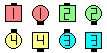Exchange The Box\$B\$NA0(B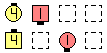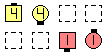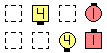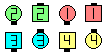\$B:8\$N(B Box1/4 \$B\$N8e(B \$B:8\$N(B Box1/2 \$B\$N8e(B \$B:8\$N(B Box3/4 \$B\$N8e(B \$B8e(B

Column \$B\$+\$i(B (\$BCf\$r8~\$\$\$?(B Centers \$B\$,%j!<%I(B):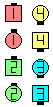Exchange The Box\$B\$NA0(B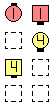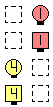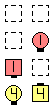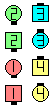\$B>e\$N(B Box1/4 \$B\$N8e(B \$B>e\$N(B Box1/2 \$B\$N8e(B \$B>e\$N(B Box3/4 \$B\$N8e(B \$B8e(B

\$B%@%s%9\$N%R%s%H(B: Exchange \$B\$N%j!<%@!<\$Oe\$2\$F(B, \$BB>\$N?M\$,\$D\$\$\$F9T\$1\$k\$h\$&\$K\$7\$^\$9!%(B

\$BExchange The Triangle [C3A].CALLERLAB definition for Exchange The BoxChoreography for Exchange The BoxComments? Questions? Suggestions?